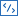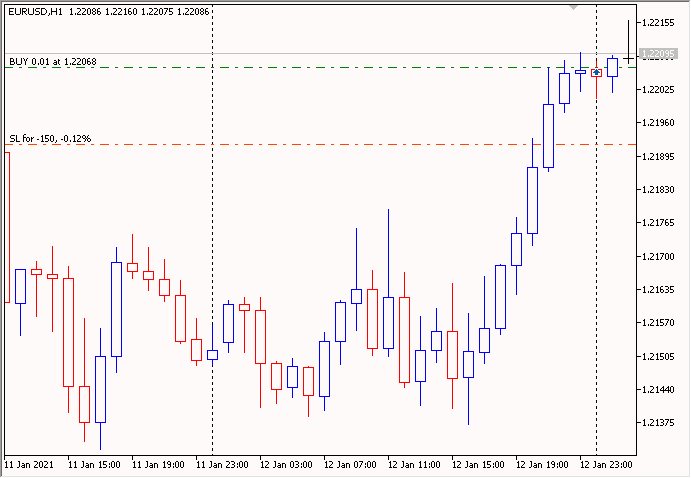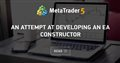# OrderSend error 4756 Invalid request

I'm sending an order but I get error 4756 with the Invalid request detail, I already tried the code in MetaQuotes it works fine but as soon as I put it in the mt5 of the broker it doesn't work, the code is as follows.

Files:
error_mql5_2.jpg  101 kb

luisfermartinezricardo123:

I'm sending an order but I get error 4756 with the Invalid request detail, I already tried the code in MetaQuotes it works fine but as soon as I put it in the mt5 of the broker it doesn't work, the code is as follows.

Please insert the code correctly: when editing a message, press the buttonand paste your code into the pop-up window

I use the 'CTrade' trading class - this avoids a lot of mistakes. Code example:

```//+------------------------------------------------------------------+
//|                                        Stop Loss Take Profit.mq5 |
//|                      https://www.mql5.com/en/users/barabashkakvn |
//+------------------------------------------------------------------+
#property version   "1.007"
#property description "Take Profit and Stop Loss - in Points (1.00055-1.00045=10 points)"
//---
CPositionInfo  m_position;                   // object of CPositionInfo class
CSymbolInfo    m_symbol;                     // object of CSymbolInfo class
//--- input parameters
input uint                 InpStopLoss             = 150;            // Stop Loss
input uint                 InpTakeProfit           = 460;            // Take Profit
input group             "Position size management (lot calculation)"
input double               InpLots                 = 0.01;           // Lots
input ulong                InpDeviation            = 10;             // Deviation, in Points (1.00045-1.00055=10 points)
input ulong                InpMagic                = 200;            // Magic number
//---
double   m_stop_loss                = 0.0;      // Stop Loss                  -> double
double   m_take_profit              = 0.0;      // Take Profit                -> double
//+------------------------------------------------------------------+
//| Expert initialization function                                   |
//+------------------------------------------------------------------+
int OnInit()
{
//--- forced initialization of variables
m_stop_loss                = 0.0;      // Stop Loss                  -> double
m_take_profit              = 0.0;      // Take Profit                -> double
//---
ResetLastError();
if(!m_symbol.Name(Symbol())) // sets symbol name
{
Print(__FILE__," ",__FUNCTION__,", ERROR: CSymbolInfo.Name");
return(INIT_FAILED);
}
RefreshRates();
//---
//---
m_stop_loss                = InpStopLoss                 * m_symbol.Point();
m_take_profit              = InpTakeProfit               * m_symbol.Point();
//--- Initialize the generator of random numbers
MathSrand(GetTickCount());
//---
return(INIT_SUCCEEDED);
}
//+------------------------------------------------------------------+
//| Expert deinitialization function                                 |
//+------------------------------------------------------------------+
void OnDeinit(const int reason)
{
//---
}
//+------------------------------------------------------------------+
//| Expert tick function                                             |
//+------------------------------------------------------------------+
void OnTick()
{
//---
if(CalculateAllPositions()==0)
{
if(!RefreshRates())
return;
//--- odd (1) - "BUY", even (2) - "SELL"
int math_rand=MathRand();
if(math_rand%2==0)
{
}
else
{
double sl=(m_stop_loss==0.0)?0.0:m_symbol.Bid()+m_stop_loss;
double tp=(m_take_profit==0.0)?0.0:m_symbol.Bid()-m_take_profit;
}
}
//---
}
//+------------------------------------------------------------------+
//| Refreshes the symbol quotes data                                 |
//+------------------------------------------------------------------+
bool RefreshRates()
{
//--- refresh rates
if(!m_symbol.RefreshRates())
{
Print(__FILE__," ",__FUNCTION__,", ERROR: ","RefreshRates error");
return(false);
}
//--- protection against the return value of "zero"
{
Print(__FILE__," ",__FUNCTION__,", ERROR: ","Ask == 0.0 OR Bid == 0.0");
return(false);
}
//---
return(true);
}
//+------------------------------------------------------------------+
//| Calculate all positions Buy and Sell                             |
//+------------------------------------------------------------------+
int CalculateAllPositions()
{
int totlal=0;
for(int i=PositionsTotal()-1; i>=0; i--)
if(m_position.SelectByIndex(i)) // selects the position by index for further access to its properties
if(m_position.Symbol()==m_symbol.Name() && m_position.Magic()==InpMagic)
totlal++;
//---
return(totlal);
}
//+------------------------------------------------------------------+
```

Result:Files:

I use the 'CTrade' trading class - this avoids a lot of mistakes. Code example:

Result:

Thank you very much, I managed to solve it. It works great for me, but I have another question, how do I get the number of the ticket that was created?

luisfermartinezricardo123 # :
Thank you very much, I managed to solve it. It works great for me, but I have another question, how do I get the number of the ticket that was created?

Clarify your question - why do you need it?

I use full control of trading orders according to the algorithm described in the article 'An attempt at developing an EA constructor'An attempt at developing an EA constructor
• www.mql5.com